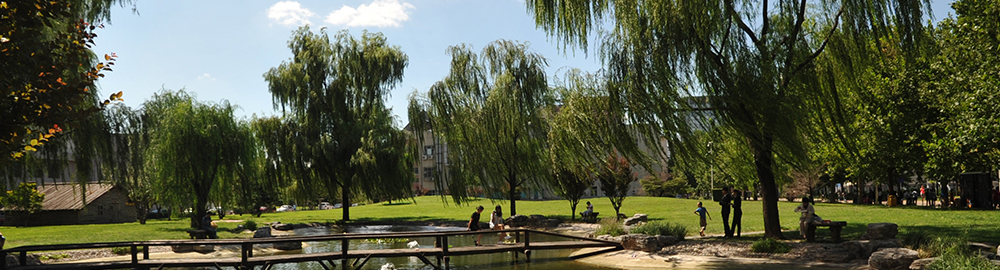## 分数域代表作

Tao, R., B. Deng, W. Q. Zhang and Y. Wang (2008). "Sampling and sampling rate conversion of band limited signals in the fractional Fourier transform domain." IEEE Transactions on Signal Processing 56(1): 158-171.

Tao, R., B. Z. Li, Y. Wang and G. K. Agarey (2008). "On Sampling of Band-Limited Signals Associated With the Linear Canonical Transform." IEEE Transactions on Signal Processing 56(11): 5454-5464.

Li, B. Z., R. Tao and Y. Wang (2007). "New sampling formulae related to linear canonical transform." Signal Processing 87(5): 983-990.

Tao, R., F. Zhang and Y. Wang (2008). "Fractional power spectrum." IEEE Transactions on Signal Processing 56(9): 4199-4206.

Deng, B., R. Tao and Y. Wang (2006). "Convolution theorems for the linear canonical transform and their applications." Science in China Series F-Information Sciences 49(5): 592-603.

Tao, R., Y. L. Li and Y. Wang (2010). "Short-Time Fractional Fourier Transform and Its Applications." IEEE Transactions on Signal Processing 58(5): 2568-2580.

Qi, L., R. Tao, S. Y. Zhou and Y. Wang (2004). "Detection and parameter estimation of multicomponent LFM signal based on the fractional Fourier transform." Science in China Series F-Information Sciences 47(2): 184-198.

Tao, R., Y. Xin and Y. Wang (2007). "Double image encryption based on random phase encoding in the fractional Fourier domain." Optics Express 15(24): 16067-16079.[code]